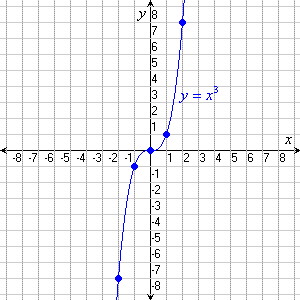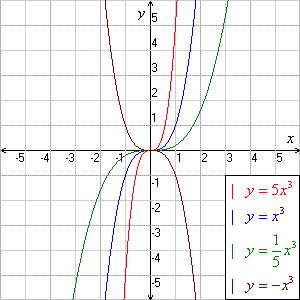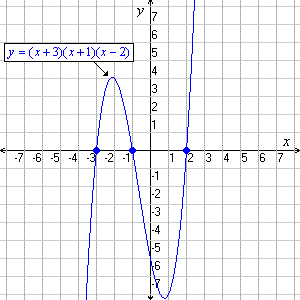# Cubic Functions

A cubic function is one in the form $f\left(x\right)=a{x}^{3}+b{x}^{2}+cx+d$ .

The "basic" cubic function, $f\left(x\right)={x}^{3}$ , is graphed below.The function of the coefficient $a$ in the general equation is to make the graph "wider" or "skinnier", or to reflect it (if negative):The constant $d$ in the equation is the $y$ -intercept of the graph.The effects of $b$ and $c$ on the graph are more complicated. However, if you can factor the right side of the equation, you can find one or more $x$ -intercepts , and use these to sketch the graph. (Some cubics, however, cannot be factored.)A cubic function may have one, two or three $x$ -intercepts, corresponding to the real roots of the related cubic equation.